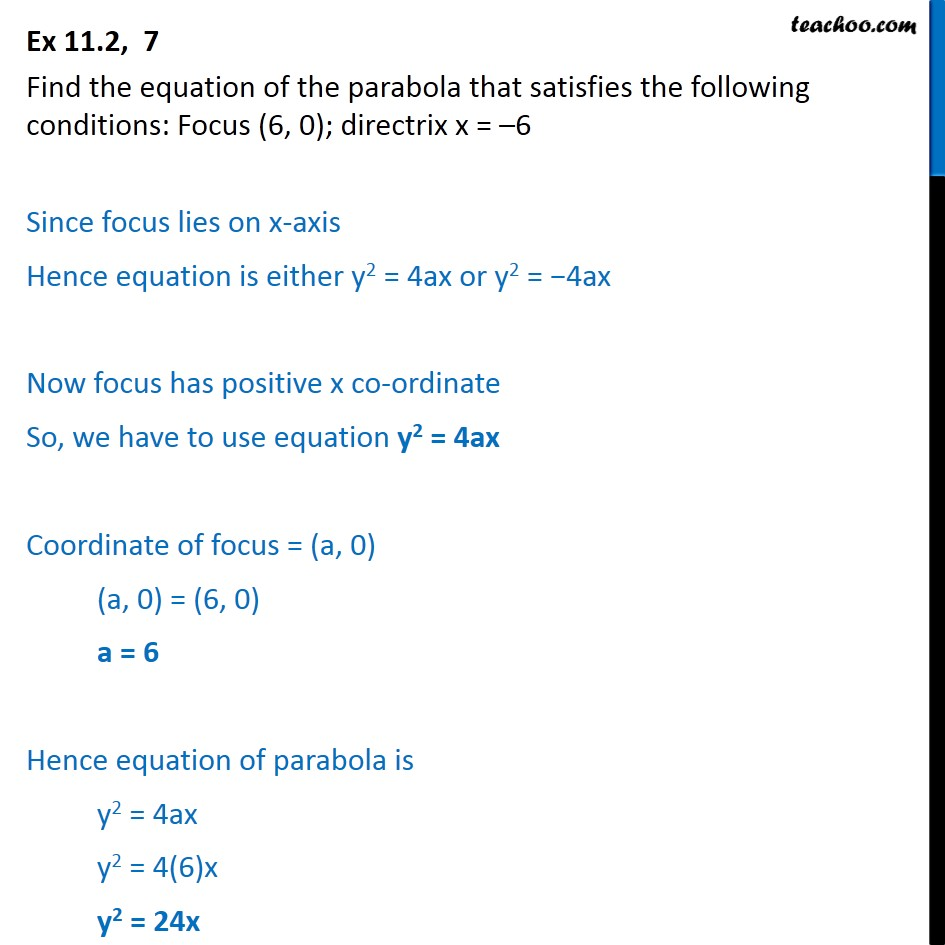Ex 10.2

Chapter 10 Class 11 Conic Sections
Serial order wiseLearn in your speed, with individual attention - Teachoo Maths 1-on-1 Class

### Transcript

Ex 10.2, 7 Find the equation of the parabola that satisfies the following conditions: Focus (6, 0); directrix x = 6 Since focus lies on x-axis Hence equation is either y2 = 4ax or y2 = 4ax Now focus has positive x co-ordinate So, we have to use equation y2 = 4ax Coordinate of focus = (a, 0) (a, 0) = (6, 0) a = 6 Hence equation of parabola is y2 = 4ax y2 = 4(6)x y2 = 24x